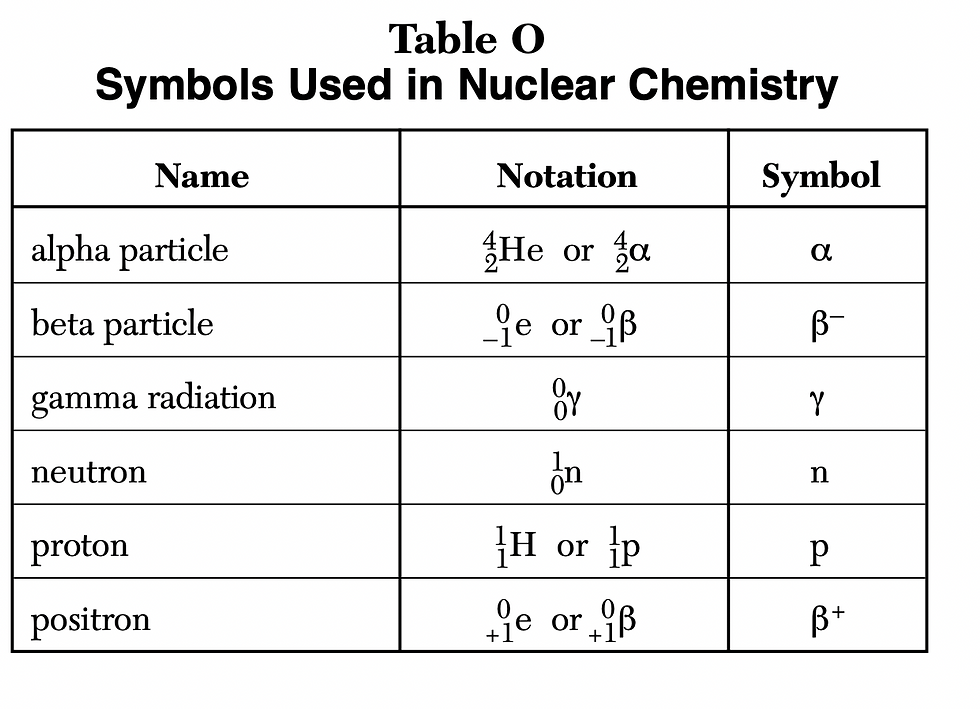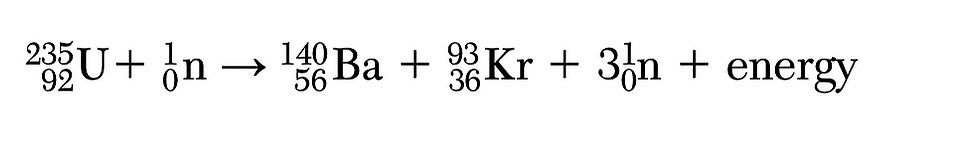top of page
Search

# Regents Chemistry Review: Nuclear Chemistry

In nuclear reactions, one element changes into a new element. This process is called transmutation.

There are two tables from the Reference Table that will be used for the nuclear chemistry topic.Table O lists nuclear particles with their respective symbols. The numbers on the top are mass numbers and the bottom ones are atomic numbers.Table N lists radiosotopes with their half lives and decay modes. β- means beta decay. β+ means positron decay. α means alpha decay mode. There might be a question on the exam asking for the decay mode of a certain radioisotope.

Half life is the amount of time it takes for the mass of the radioisotope to become one half of the original amount. For example, C-14 has a half life of 5715 years. It will take 5715years for 100 grams of C-14 to become 50 grams.

Solving Nuclear Reaction

To find an unknown in the nuclear reaction, we will solve for the mass and atomic number.

The sum of masses (top numbers) to the left of arrow (reactants) will be equal to the sum of the masses of products (to the right of the arrow).

The sum of atomic numbers (on the bottom)to the left of arrow (reactants) will be equal to the sum of the atomic numbers of products (to the right of the arrow).

Once we know the atomic number, we can search for that atomic number in the Periodic Table to find the element. If the unknown is a particle, we can use Table O to identify it.

Example: Given the equation representing a nuclear reaction:Solution: To find X, we must find the mass number and the atomic number. To find mass number we add masses(on top) on the reactant side and make it equal to the sum of masses on the product side. We do 1+x = 6+4 x=9. Mass must be 9, eliminating choices 3 and 4. Next we do the same for atomic number (bottom numbers). 1+y = 3+2 y=4. The atomic number is 4. Next, we are going to check which element has an atomic number of 4 on the Periodic Table. Be has a mass number of 4.

Natural (spontaneous decay) Versus Artificial Transmutation

Natural transmutation (or spontaneous decay): We will see only one element to the left of the arrow (reactant side).

Artificial Transmutation: We will see an element and a particle (such as alpha particle or He) to the left of the arrow (reactant side).

Nuclear Reactions

Fusion: light nuclei come together to form a heavier nucleus.

Example:Fission: bombardment of a large atom such as uranium with a neutron.

Example:Iodine-131 = treatment of thyroid disorders

Iodine-123 = detection of thyroid disorders

Carbon-14 = used as a tracer, to follow the course of a chemical reaction. It is also used for dating lining materials (figuring out the age of wood, bone etc)

Cobalt-60 = sterilizing medical equipment

Uranium-238 = geological dating (figuring out the age of rocks)

Facts to remember

• The ratio of neutrons to protons determine whether nuclei are stable or not.

• Gamma rays have highest penetrating power and lowest ionizing power.

• Alpha rays have lowest penetrating power and higher ionizing power.

• Nuclear reaction such as fission and fusion convert mass into energy. These reactions produce much more energy than chemical reactions.

Prepare for the Chemistry Regents Exam: HERE